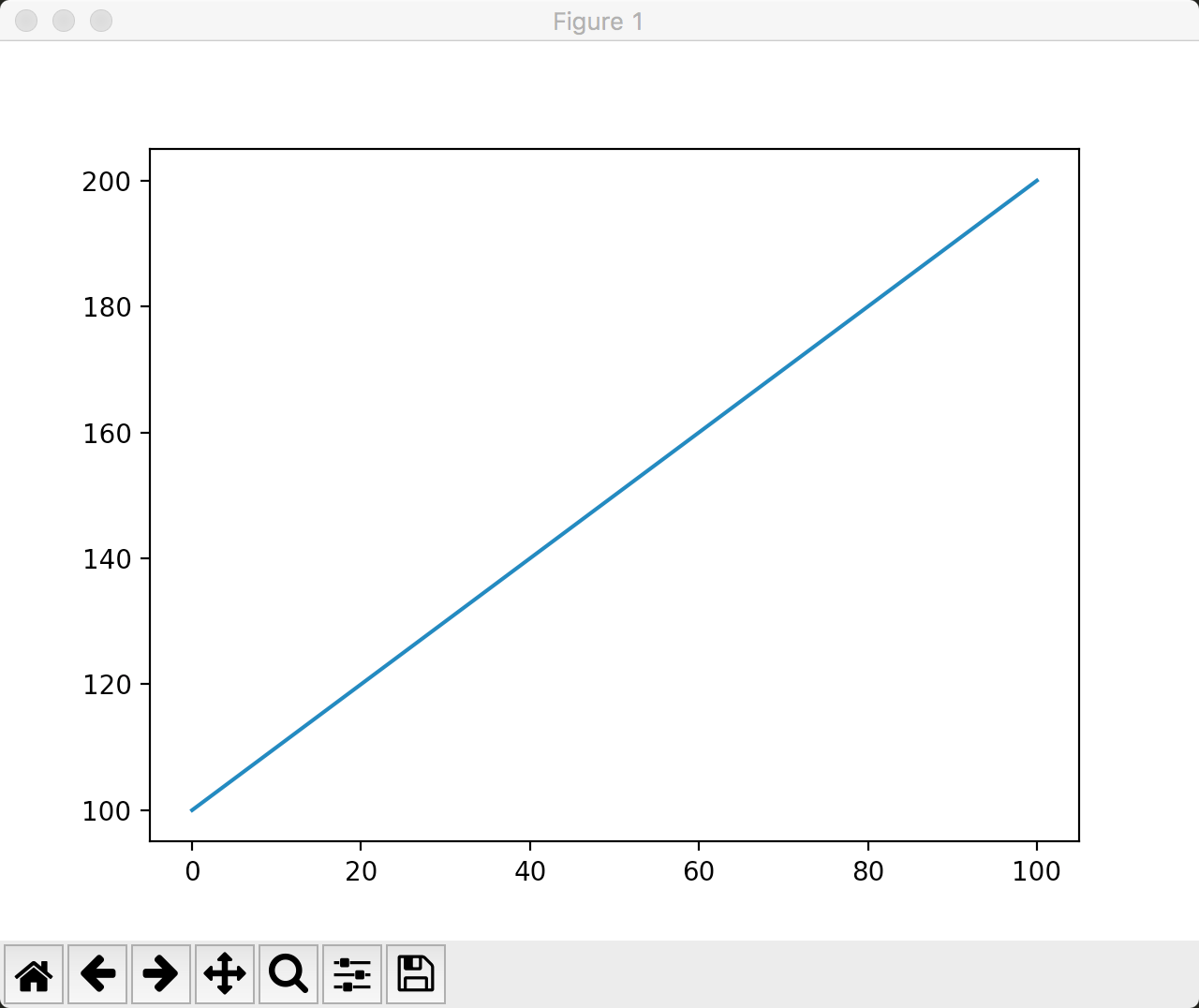# Matplotlib入门

via: 保罗的酒吧 https://paul.pub/matplotlib-basics/

## 入门代码示例

``````import matplotlib.pyplot as plt
import numpy as np

data = np.arange(100,201)
plt.plot(data)
plt.show()
````````````x = np.linspace(0, 2*np.pi,50)  # 50个元素均匀分布在[0,2pi]上
plt.plot(x, np.sin(x))
plt.show()
``````## 一次绘制多个图形

### 多个figure

``````import matplotlib.pyplot as plt
import numpy as np

data = np.arange(100,201)
plt.plot(data)

data2 = np.arange(200,301)
plt.figure()
plt.plot(data2)

plt.show()
``````### 多个subplot

``````import matplotlib.pyplot as plt
import numpy as np

data = np.arange(100,201)
plt.subplot(2,1,1)
plt.plot(data)

data2 = np.arange(200,301)
plt.subplot(2,1,2)
plt.plot(data2)

plt.show()
``````subplot函数的参数不仅仅支持上面这种形式，还可以将三个整数（10之内的）合并一个整数。例如：2, 1, 1可以写成211，2, 1, 2可以写成212。

subplot函数的详细说明参见这里：matplotlib.pyplot.subplot

### 在一张图上绘制多个数据集

``````x = np.linspace(0, 2*np.pi，150)
plt.plot(x, np.sin(x), x, np.sin(2*x), x, np.sin(3*x))
plt.show()
````````````x = np.linspace(0, 2*np.pi, 50)
plt.plot(x, np.sin(x), 'r-^', x, np.sin(2*x), 'g--')
plt.show()
``````## 常用图形实例

Matplotlib可以生成非常多的图形式样，多到令人惊叹的地步。

## 线性图

``````import matplotlib.pyplot as plt
plt.plot([1,2,3],[3,6,9],'r')  # red
plt.plot([1,2,3],[2,4,9],':g')  # :(点) green

plt.show()
``````### 散点图

`scatter`函数用来绘制散点图。同样，这个函数也需要两组配对的数据指定x和y轴的坐标。下面是一段代码示例：

``````N = 20  # 点的个数

plt.scatter(np.random.rand(N)*100,
np.random.rand(N)*100,
c='r',s=100,alpha=0.5)  # c：点的颜色  s：点的大小  alpha：点的透明度

plt.scatter(np.random.rand(N)*100,
np.random.rand(N)*100,
c='g',s=200,alpha=0.5)

plt.scatter(np.random.rand(N)*100,
np.random.rand(N)*100,
c='b',s=300,alpha=0.5)

plt.show()
``````### 饼状图

`pie`函数用来绘制饼状图。饼状图通常用来表达集合中各个部分的百分比。

In :

``````labels = ['Mon','Tue','Wed','Thu','Fri','Sat','Sun']

data = np.random.rand(7)*100

plt.pie(data, labels=labels, autopct='%1.1f%%')  # autopct 指定数值的精度
plt.axis('equal')  # 设置坐标轴大小一致
plt.legend()  # 指明要绘制图例

plt.show()
``````### 条形图

`bar`函数用来绘制条形图。条形图常常用来描述一组数据的对比情况，例如：一周七天，每天的城市车流量。

``````N = 7

x = np.arange(7)
data = np.random.randint(low=0, high=100, size=N)  # 生成7个0-100的随机数
colors = np.random.rand(N*3).reshape(N,-1)  # 先生成21（N x 3）个随机数，然后将它们组装成7行，那么每行就是三个数，这对应了颜色的三个组成部分。
labels = ['Mon','Tue','Wed','Thu','Fri','Sat','Sun']

plt.title('Weekday Data')
plt.bar(x, data, alpha=0.8, color=colors, tick_label=labels)
plt.show()
``````### 直方图

`hist`函数用来绘制直方图。直方图看起来是条形图有些类似。但它们的含义是不一样的，直方图描述了数据中某个范围内数据出现的频度。

``````data = [np.random.randint(0,n,n) for n in [3000,4000,5000]]
labels = ['3K','4K','5K']
bins = [0,100,500,1000,2000,3000,4000,5000]

plt.hist(data, bins=bins, label=labels)
plt.legend()
plt.show()
``````– 第一个数组包含了3000个随机数，这些随机数的范围是 [0, 3000)
– 第二个数组包含了4000个随机数，这些随机数的范围是 [0, 4000)
– 第三个数组包含了5000个随机数，这些随机数的范围是 [0, 5000)
bins数组用来指定我们显示的直方图的边界，即：[0, 100) 会有一个数据点，[100, 500)会有一个数据点，以此类推。所以最终结果一共会显示7个数据点。同样的，我们指定了标签和图例。